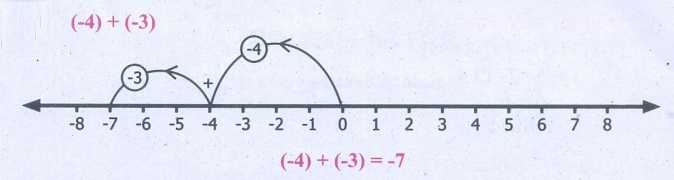Home | | Maths 7th Std | Addition of Integers

## Chapter: 7th Maths : Term 1 Unit 1 : Number System

The number line is a simple tool to visualise addition of integers. Let us do an activity with the number line.

The number line is a simple tool to visualise addition of integers. Let us do an activity with the number line.

Imagine that the number line is a road with markers on it. We are allowed to step forward or backward on this road. One step taken is equal to one unit of number. Initially we start at zero and face positive direction. We step forward for positive integers and backward for negative integers. We maintain the same positive direction for addition operation.To add (+5) and (–3), We start at zero facing positive direction and move five steps forward to represent (+5). Since the operation is addition we maintain the same direction and move three units backward to represent (–3). We land at +2. So, (+5)+(–3)=2. This is shown in Fig.1.5.Proceeding in the same way let us try another example. Find the sum of (–6) and (–4). We start at zero facing positive direction continuing in the same direction and move 6 units backward to represent (–6) and in the same direction move 4 units backward to represent (–4). We land at (–10).

Therefore, (–6)+(–4) = –10Try this

Find the value of the following using the number line activity:

(i) (–4) + (+3)–4 + 3 = –1

(ii) (–4) + (–3)(–4) +(–3) = –7

(iii) (+4) + (–3)(+4) + (–3) = +l

Activity

There are two bowls with tokens of two different colours brown and pink. Let us denote one brown token by (+1) and one pink token by (–1). A pair of tokens, brown (+1) and pink (–1), will denote zero [1+(–1)=0]To add two integers, we pick the required number of tokens and form possible zero pairs. The remaining number of tokens left after pairing is the sum of the two integers.To add (–7) and (+5) we pick 7 pink tokens and 5 brown tokens. We form zero pairs from the tokens as above. We can form 5 zero pairs. Then we are left with 2 pink tokens. Hence, ( 7) + (+5) = −2 .To add (–3) and (–4) we pick 3 pink tokens first and 4 pink tokens later. The total number of tokens is 7 pink tokens. There are no zero pairs. So, the sum of (–3) and (–4) is (–7). Teacher can give different integers and ask them to add using tokens.

Note

1. When we add two integers of the same sign the sum will also be an integer of the same sign. When we add two integers of different sign, the sum will be the difference between the two integers and have the sign of the integer with greater value.

1. The integer wihtout sign represents positive interger.

Example 1.1

Add the following integers using number line (i) 10 and –15 (ii) –7 and –9

Solution

Let us add the intergers using number line

(i) 10 and –15On the number line we first start at zero facing positive direction and move 10 steps forward, reaching 10. Then we move 15 steps backward to represent –15 and reach at –5. Thus, we get 10 + (–15) = –5.

(ii) –7 and –9On the number line we first start at zero facing positive direction and move 7 steps backward, reaching –7. Then we move 9 steps backward to represent –9 and reach at –16. Thus, we get (–7) + (–9) = –16.

Example 1.2

Add (i) (–40) and (30) (ii) 60 and (–50)

Solution

(i) (–40) and (30)

–40 + 30 = –10

(ii) 60 and (–50)

60+(–50)=60–50=10

Example 1.3

Add: (i) (–70) and (–12) (ii) 103 and 39.

Solution

(i) (–70) + (–12) = –70 – 12 = – 82

(ii) 103 + 39 = 142

Example 1.4

A submarine is at 32 feet below the sea level. Then it moves up 8 feet. Find the depth of the submarine.

Solution

A submarine is 32 feet below sea level.

Therefore, it is represented by –32

Next it moves up 8 feet.

Moves above is represented as +8

The depth of the submarine = –32 + 8 = –24

Therefore, the submarine is located at 24 feet below the sea level.

Example 1.5

Sita saved ₹ 225.00 and she has spent ₹ 400 on credit basis for the purchase of  stationery. Find her due amount.

Solution

The amount Sita has ₹ 225

The amount spent for stationery on credit =₹400

The due amount to be paid = ₹225 – ₹400 = –₹175

Therefore, Sita has to pay ₹ 175

Example 1.6

From the ground floor a man went up six floors and came down six floors. In which floor is he now?

Solution

Starting point = Ground floor

Number of floors climbed up = +6

Number of floors climbed down = –6

Now the landing point = +6 – 6 = 0 (ground floor)

Tags : Number System | Term 1 Chapter 1 | 7th Maths , 7th Maths : Term 1 Unit 1 : Number System
Study Material, Lecturing Notes, Assignment, Reference, Wiki description explanation, brief detail
7th Maths : Term 1 Unit 1 : Number System : Addition of Integers | Number System | Term 1 Chapter 1 | 7th Maths